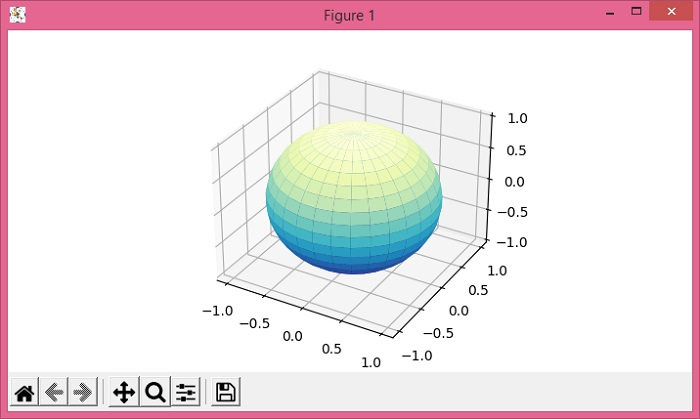# Plotting points on the surface of a sphere in Python's Matplotlib

To plot points on the surface of a sphere in Python, we can use plot_surface() method.

## Steps

• Create a new figure or activate an existing figure using figure() method.

• Add a set of subplots using add_subplot() method with 3d projection.

• Initialize a variable, r.

• Get the theta value for spherical points and x, y, and z data points using numpy.

• Plot the surface using plot_surface() method.

• To display the figure, use show() method.

## Example

import matplotlib.pyplot as plt
import numpy as np
plt.rcParams["figure.figsize"] = [7.00, 3.50]
plt.rcParams["figure.autolayout"] = True
fig = plt.figure()
plt.show()